# ISEE Upper Level Quantitative : How to find the length of the hypotenuse of a right triangle : Pythagorean Theorem

## Example Questions

← Previous 1

### Example Question #1 : Right Triangles

What is the hypotenuse of a right triangle with sides 5 and 8?

5√4

12

√89

15

√89

Explanation:

Because this is a right triangle, we can use the Pythagorean Theorem which says a2 + b2 = c2, or the squares of the two sides of a right triangle must equal the square of the hypotenuse. Here we have a = 5 and b = 8.

a2 + b2 = c2

52 + 82 = c2

25 + 64 = c2

89 = c2

c = √89

### Example Question #1 : How To Find The Length Of The Hypotenuse Of A Right Triangle : Pythagorean Theorem

Which is the greater quantity?

(a) The hypotenuse of a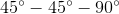right triangle with a leg of length 20

(b) The hypotenuse of a right triangle with legs of length 19 and 21

It is impossible to tell from the information given

(a) and (b) are equal

(b) is greater

(a) is greater

(b) is greater

Explanation:

The hypotenuses of the triangles measure as follows:

(a)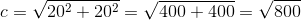(b)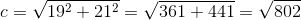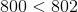, so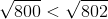, making (b) the greater quantity

### Example Question #3 : Right Triangles

Which is the greater quantity?

(a) The hypotenuse of a right triangle with legsand.

(b) The hypotenuse of a right triangle with legsand.

(a) and (b) are equal.

It is impossible to tell from the information given.

(b) is greater.

(a) is greater.

(a) is greater.

Explanation:

The hypotenuses of the triangles measure as follows:

(a)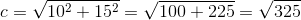(b)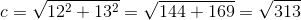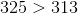, so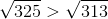, making (a) the greater quantity.

### Example Question #4 : Right Triangles

A right triangle has a leg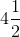feet long and a hypotenuse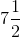feet long. Which is the greater quantity?

(a) The length of the second leg of the triangle

(b) 60 inches

It is impossible to tell from the information given.

(b) is greater.

(a) and (b) are equal.

(a) is greater.

(a) is greater.

Explanation:

The length of the second leg can be calculated using the Pythagorean Theorem. Set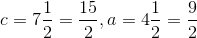: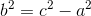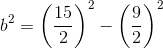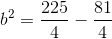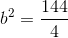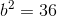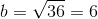The second leg therefore measures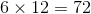inches.

### Example Question #5 : Right Triangles

What is the hypotenuse of a right triangle with sides 9 inches and 12 inches?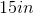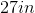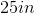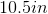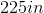Explanation:

Since we're dealing with right triangles, we can use the Pythagorean Theorem (). In this formula, a and b are the sides, while c is the hypotenuse. The hypotenuse of a right triangle is the longest side and the side that is opposite the right angle. Now, we can plug into our formula, which looks like this: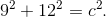We simplify and get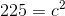. At this point, isolate c. This means taking the square root of both sides so that your answer is 15in.

### Example Question #6 : Right Triangles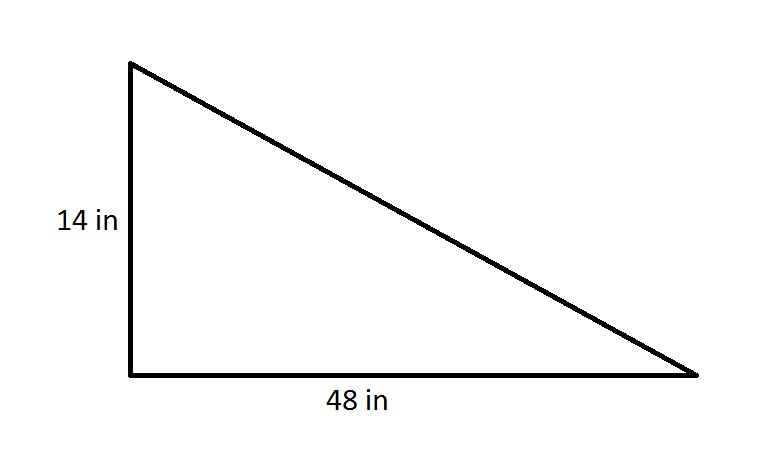The perimeter of a regular pentagon is 75% of that of the triangle in the above diagram. Which is the greater quantity?

(A) The length of one side of the pentagon

(B) One and one-half feet

(A) and (B) are equal

It is impossible to determine which is greater from the information given

(B) is greater

(A) is greater

(B) is greater

Explanation:

By the Pythagorean Theorem, the hypotenuse of the right triangle is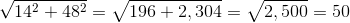inches, making its perimeter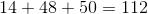inches.

The pentagon in question has sides of length 75% of 112, or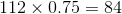.

Since a pentagon has five sides of equal length, each side will have measure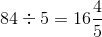inches.

One and a half feet are equivalent to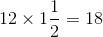inches, so (B) is the greater quantity.

### Example Question #7 : Right Triangles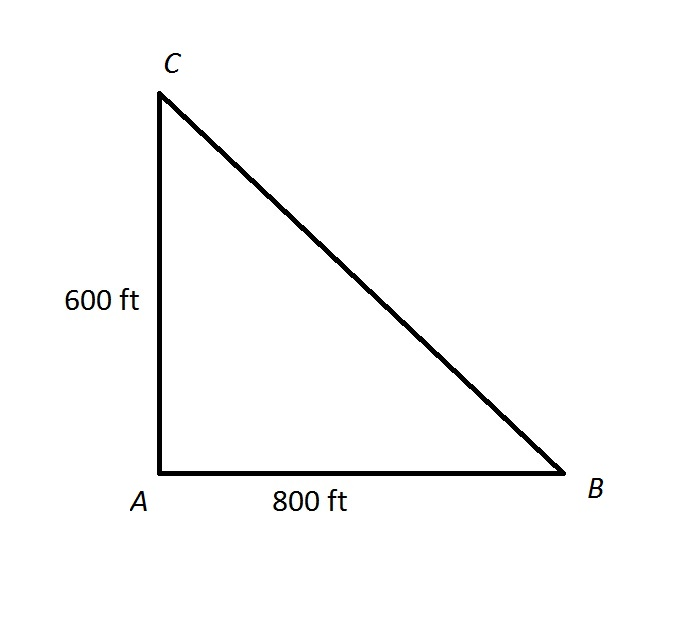The track at Gauss High School is unusual in that it is shaped like a right triangle, as shown above.

Cary decides to get some exercise by running from point A to point B, then running half of the distance from point B to point C.

Which is the greater quantity?

(A) The distance Cary runs

(B) One-fourth of a mile

(B) is greater

It is impossible to determine which is greater from the information given

(A) and (B) are equal

(A) is greater

(B) is greater

Explanation:

By the Pythagorean Theorem, the distance from B to C is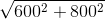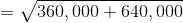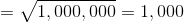feet

Cary runs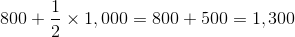feet

Since 5,280 feet make a mile, one-fourth of a mile is equal to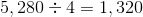feet.

(B) is greater

### Example Question #8 : Right Triangles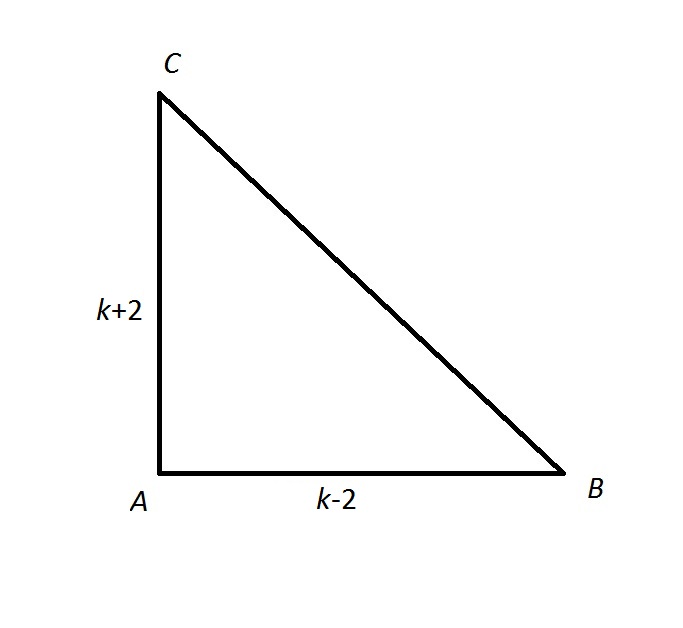Give the length of the hypotenuse of the above right triangle in terms of.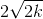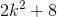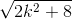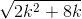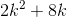Explanation:

If we letbe the length of the hypotenuse, then by the Pythagorean theorem,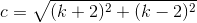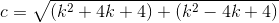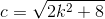### Example Question #9 : Right Triangles

In Square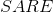is the midpoint of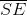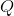is the midpoint of, and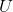is the midpoint of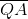. Construct the line segments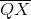and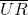.

Which is the greater quantity?

(a)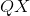(b)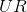It cannot be determined which of (a) and (b) is greater

(a) and (b) are equal

(a) is the greater quantity

(b) is the greater quantity

(b) is the greater quantity

Explanation:

The figure referenced is below: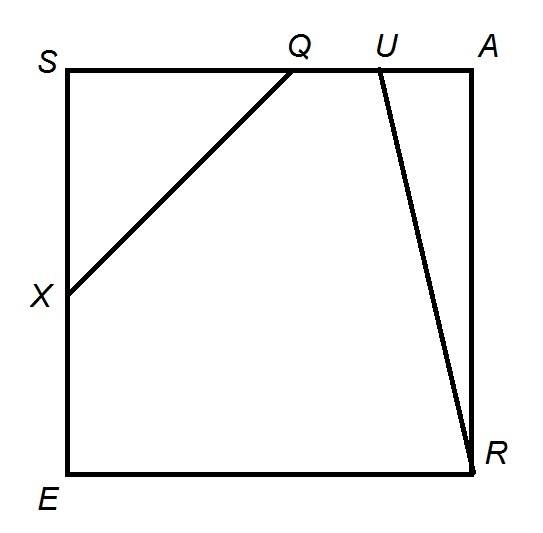For the sake of simplicity, assume that the square has sides of length 4. The following reasoning is independent of the actual lengths, and the reason for choosing 4 will become apparent in the explanation.andare midpoints of their respective sides, so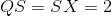, makingthe hypotenuse of a triangle with legs of length 2 and 2. Therefore,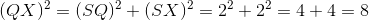.

Also,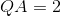, and sinceis the midpoint of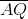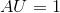.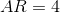, makingthe hypotenuse of a triangle with legs of length 1 and 4. Therefore,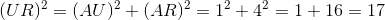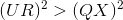, so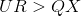### Example Question #10 : Right Triangles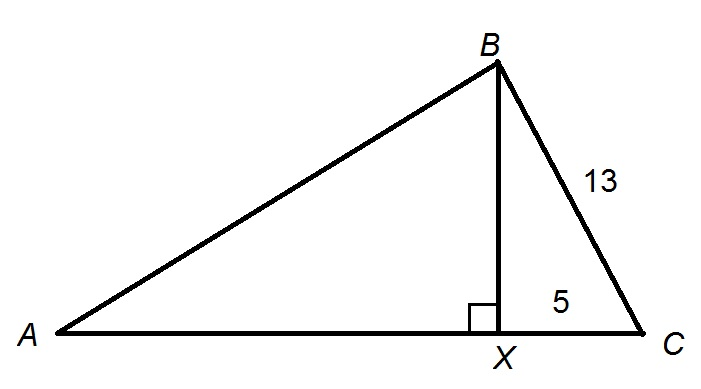Figure NOT drawn to scale.

In the above figure,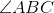is a right angle.

What is the length of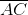?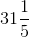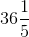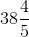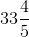Explanation:

The altitude of a right triangle from the vertex of its right angle divides the triangle into two smaller triangles each similar to the larger triangle. In particular,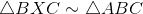.

Their corresponding sides are in proportion, so, setting the ratios of the hypotenuses to the short legs equal to each other,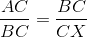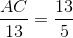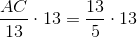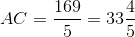← Previous 1